Most Affordable JEE | NEET | 8,9,10 Preparation by Kota's Top IITian Doctor Faculties

# NCERT Solutions for Class 12 Chemistry Chapter 7 The P Block Elements PDF - eSaral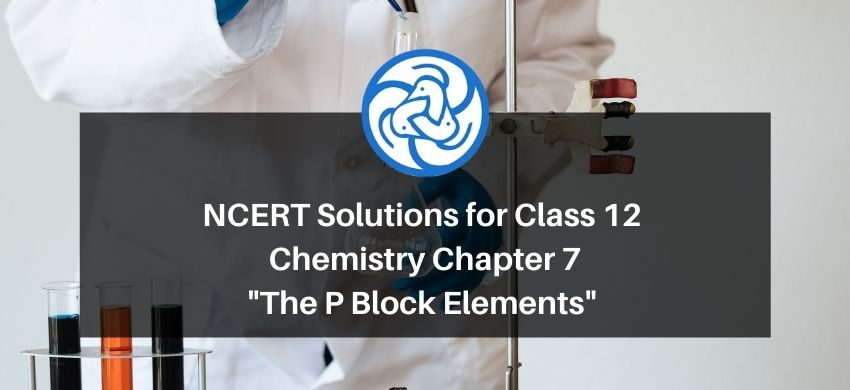# NCERT Solutions for Class 12 Chemistry Chapter 7 The P Block Elements PDF

Hey, are you a class 12 student and looking for ways to download NCERT Solutions for Class 12 Chemistry Chapter 7 The P Block Elements PDF? If yes. Then read this post till the end.

In this article, we have listed NCERT Solutions for Class 12 Chemistry Chapter 7 The P Block Elements in PDF that are prepared by Kota’s top IITian Faculties by keeping Simplicity in mind.

If you want to learn and understand class 12 Chemistry Chapter 7 "The P Block Elements" in an easy way then you can use these solutions PDF.

NCERT Solutions helps students to Practice important concepts of subjects easily. Class 12 Chemistry solutions provide detailed explanations of all the NCERT questions that students can use to clear their doubts instantly.

If you want to score high in your class 12 Chemistry Exam then it is very important for you to have a good knowledge of all the important topics, so to learn and practice those topics you can use eSaral NCERT Solutions.

In this article, we have listed NCERT Solutions for Class 12 Chemistry Chapter 7 The P Block Elements PDF that you can download to start your preparations anytime.

So, without wasting more time Let’s start.

### Download NCERT Solutions for Class 12 Chemistry Chapter 7 The P Block Elements PDF

Question 1: Why are pentahalides more covalent than trihalides?

Solution. In pentahalides, the oxidation state is $+5$ and in trihalides, the oxidation state is $+3 .$ Since the metal ion with a high charge has more polarizing power, pentahalides are more covalent than trihalides.

Question 2: Why is $\mathrm{BiH}_{3}$ the strongest reducing agent amongst all the hydrides of

Group 15 elements?

Solution. As we move down a group, the atomic size increases and the stability of the hydrides of group 15 elements decreases. Since the stability of hydrides decreases on moving from $\mathrm{NH}_{3}$ to $\mathrm{BiH}_{3}$, the reducing character of the hydrides increases on moving from $\mathrm{NH}_{3}$ to $\mathrm{BiH}_{3}$.

Question 3: Why is $\mathrm{N}_{2}$ less reactive at room temperature?

Solution. The two $\mathrm{N}$ atoms in $\mathrm{N}_{2}$ are bonded to each other by very strong triple covalent bonds. The bond dissociation energy of this bond is very high. As a result, $N_{2}$ is less reactive at room temperature.

Question 4: Mention the conditions required to maximise the yield of ammonia.

Solution. Ammonia is prepared using the Haber's process. The yield of ammonia can be maximized under the following conditions:

(i) High pressure $(\sim 200 \mathrm{~atm})$

(ii) A temperature of $\sim 700 \mathrm{~K}$

(iii) Use of a catalyst such as iron oxide mixed with small amounts of $\mathrm{K}_{2} \mathrm{O}$ and $\mathrm{Al}_{2} \mathrm{O}_{3}$

Question $5$ : How does ammonia react with a solution of $\mathrm{Cu}^{2+}$ ?

Solution. $\mathrm{NH}_{3}$ acts as a Lewis base. It donates its electron pair and forms a linkage with metal ion.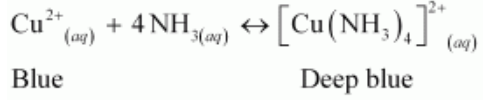Question $6$ : What is the covalence of nitrogen in $\mathrm{N}_{2} \mathrm{O}_{5} ?$

Solution.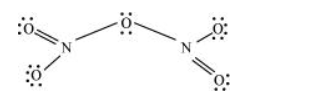From the structure of $\mathrm{N}_{2} \mathrm{O}_{5}$, it is evident that the covalence of nitrogen is $4 .$

Question 7: Bond angle in $\mathrm{PH}_{4}^{+}$is higher than that in $\mathrm{PH}_{3}$. Why?

Solution. In $\mathrm{PH}_{3}, \mathrm{P}$ is $s p^{3}$ hybridized. Three orbitals are involved in bonding with three hydrogen atoms and the fourth one contains a lone pair. As lone pair-bond pair repulsion is stronger than bond pair-bond pair repulsion, the tetrahedral shape associated with $s p^{3}$ bonding is changed to pyramidal. $\mathrm{PH}_{3}$ combines with a proton to form $\mathrm{PH}_{4}^{+}$in which the lone pair is absent. Due to the absence of lone pair in $\mathrm{PH}_{4}^{+}$, there is no lone pair-bond pair repulsion. Hence, the bond angle in $\mathrm{PH}_{4}^{+}$is higher than the bond angle in $\mathrm{PH}_{3}$.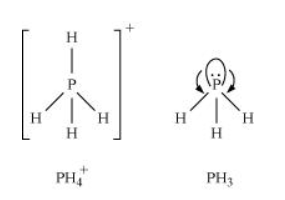Question 8: What happens when white phosphorus is heated with concentrated $\mathrm{NaOH}$ solution in an inert atmosphere of $\mathrm{CO}_{2} ?$

Solution. White phosphorous dissolves in boiling $\mathrm{NaOH}$ solution (in a $\mathrm{CO}_{2}$ atmosphere) to give phosphine, $\mathrm{PH}_{3}$.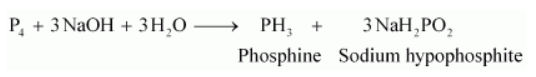Question 9: What happens when $\mathrm{PCl}_{5}$ is heated?

Solution. All the bonds that are present in $\mathrm{PCl}_{5}$ are not similar. It has three equatorial and two axial bonds. The equatorial bonds are stronger than the axial ones. Therefore, when $\mathrm{PCl}_{5}$ is heated strongly, it decomposes to form $\mathrm{PCl}_{3}$

$\mathrm{PCl}_{5} \stackrel{\text { heat }}{\longrightarrow} \mathrm{PCl}_{3}+\mathrm{Cl}_{2}$

Question 10: Write a balanced equation for the hydrolytic reaction of $\mathrm{PCl}_{5}$ in heavy water.

Solution. $\mathrm{PCl}_{5}+\mathrm{D}_{2} \mathrm{O} \longrightarrow \mathrm{POCl}_{3}+2 \mathrm{DCl}_{2}$

$\mathrm{POCl}_{3}+3 \mathrm{D}_{2} \mathrm{O} \longrightarrow \mathrm{D}_{3} \mathrm{PO}_{4}+3 \mathrm{DCl}$

Therefore, the net reaction can be written as

$\mathrm{PCl}_{5}+4 \mathrm{D}_{2} \mathrm{O} \longrightarrow \mathrm{D}_{3} \mathrm{PO}_{4}+5 \mathrm{DCl}$

Question $11$ : What is the basicity of $\mathrm{H}_{3} \mathrm{PO}_{4} ?$

Solution. $\mathrm{H}_{3} \mathrm{PO}_{4}$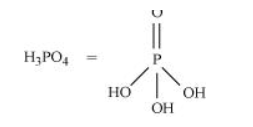Since there are three OH groups present in $\mathrm{H}_{3} \mathrm{PO}_{4}$, its basicity is three i.e., it is a tribasic acid.

Question 12: What happens when $\mathrm{H}_{3} \mathrm{PO}_{3}$ is heated?

Solution. $\mathrm{H}_{3} \mathrm{PO}_{3}$, on heating, undergoes disproportionation reaction to form $\mathrm{PH}_{3}$ and $\mathrm{H}_{3} \mathrm{PO}_{4} .$ The oxidation numbers of $\mathrm{P}$ in $\mathrm{H}_{3} \mathrm{PO}_{3}, \mathrm{PH}_{3}$, and $\mathrm{H}_{3} \mathrm{PO}_{4}$ are $+3,-3$, and $+5$ respectively. As the oxidation number of the same element is decreasing and increasing during a particular reaction, the reaction is a disproportionation reaction.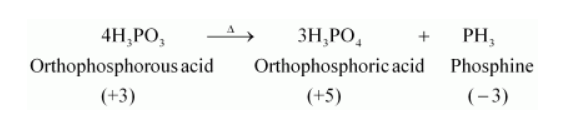Question 13: List the important sources of sulphur.

Solution. Sulphur mainly exists in combined form in the earth's crust primarily as sulphates [gypsum $\left(\mathrm{CaSO}_{4} \cdot 2 \mathrm{H}_{2} \mathrm{O}\right)$, Epsom salt $\left(\mathrm{MgSO}_{4} \cdot 7 \mathrm{H}_{2} \mathrm{O}\right)$, baryte $\left.\left(\mathrm{BaSO}_{4}\right)\right]$ and sulphides [(galena (PbS), zinc blends $(\mathrm{ZnS})$, copper pyrites $\left.\left(\mathrm{CuFeS}_{2}\right)\right]$.

Question $14$ : Write the order of thermal stability of the hydrides of Group 16 elements.

Solution. The thermal stability of hydrides decreases on moving down the group. This is due to a decrease in the bond dissociation enthalpy $(\mathrm{H}-\mathrm{E})$ of hydrides on moving down the group.

Therefore,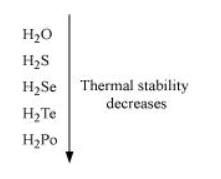Question $15$ : Why is $\mathrm{H}_{2} \mathrm{O}$ a liquid and $\mathrm{H}_{2} \mathrm{~S}$ a gas?

Solution. $\mathrm{H}_{2} \mathrm{O}$ has oxygen as the central atom. Oxygen has smaller size and higher electronegativity as compared to sulphur. Therefore, there is extensive hydrogen bonding in $\mathrm{H}_{2} \mathrm{O}$, which is absent in $\mathrm{H}_{2}$ S. Molecules of $\mathrm{H}_{2} \mathrm{~S}$ are held together only by weak van der Waal's forces of attraction.

Hence, $\mathrm{H}_{2} \mathrm{O}$ exists as a liquid while $\mathrm{H}_{2} \mathrm{~S}$ as a gas.

Question 16: Which of the following does not react with oxygen directly?

$\mathrm{Zn}, \mathrm{Ti}, \mathrm{Pt}, \mathrm{Fe}$

Solution. $\mathrm{Pt}$ is a noble metal and does not react very easily. All other elements, $\mathrm{Zn}, \mathrm{Ti}, \mathrm{Fe}$, are quite reactive. Hence, oxygen does not react with platinum (Pt) directly.

Question 17: Complete the following reactions:

(i) $\mathrm{C}_{2} \mathrm{H}_{4}+\mathrm{O}_{2} \rightarrow$

(ii) $4 \mathrm{Al}+3 \mathrm{O}_{2} \rightarrow$

Solution.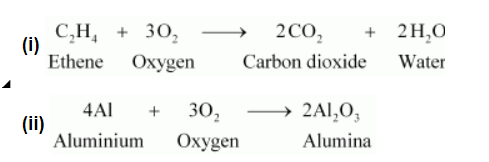Question 18: Why does $\mathrm{O}_{3}$ act as a powerful oxidising agent?

Solution. Ozone is not a very stable compound under normal conditions and decomposes readily on heating to give a molecule of oxygen and nascent oxygen. Nascent oxygen, being a free radical, is very reactive.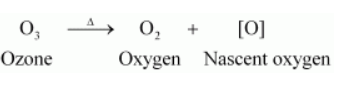Therefore, ozone acts as a powerful oxidising agent.

Question 19: How is $\mathrm{O}_{3}$ estimated quantitatively?

Solution. Quantitatively, ozone can be estimated with the help of potassium iodide. When ozone is made to react with potassium iodide solution buffered with a borate buffer (pH 9.2), iodine is liberated. This liberated iodine can be titrated against a standard solution of sodium thiosulphate using starch as an indicator. The reactions involved in the process are given below.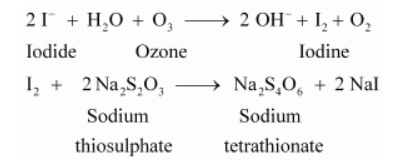Question $20$ : What happens when sulphur dioxide is passed through an aqueous solution of $\mathrm{Fe}(\mathrm{III})$ salt?

Solution. $\mathrm{SO}_{2}$ acts as a reducing agent when passed through an aqueous solution containing $\mathrm{Fe}(\mathrm{III})$ salt. It reduces $\mathrm{Fe}(\mathrm{III})$ to $\mathrm{Fe}(\mathrm{II})$ i.e., ferric ions to ferrous ions.

$2 \mathrm{Fe}^{3+}+\mathrm{SO}_{2}+2 \mathrm{H}_{2} \mathrm{O} \longrightarrow 2 \mathrm{Fe}^{2+}+\mathrm{SO}_{4}^{2-}+4 \mathrm{H}^{+}$

Question 21: Comment on the nature of two $\mathrm{S}-\mathrm{O}$ bonds formed in $\mathrm{SO}_{2}$ molecule. Are the two $\mathrm{S}-\mathrm{O}$ bonds in this molecule equal?

Solution. The electronic configuration of $S$ is $1 s^{2} 2 s^{2} 2 p^{6} 3 s^{2} 3 p^{4}$.

During the formation of $S O_{2}$, one electron from $3 p$ orbital goes to the $3 d$ orbital and $S$ undergoes $s p^{2}$ hybridization. Two of these orbitals form sigma bonds with two oxygen atoms and the third contains a lone pair. $p$-orbital and $d$-orbital contain an unpaired electron each. One of these electrons forms pा- pit bond with one oxygen atom and the other forms pm- dit bond with the other oxygen. This is the reason $\mathrm{SO}_{2}$ has a bent structure. Also, it is a resonance hybrid of structures I and II.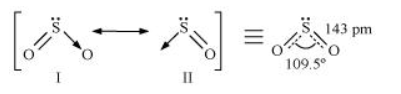Both $S-O$ bonds are equal in length $(143 \mathrm{pm})$ and have a multiple bond character.

Question 22: How is the presence of $\mathrm{SO}_{2}$ detected?

Solution. $\mathrm{SO}_{2}$ is a colourless and pungent smelling gas.

It can be detected with the help of potassium permanganate solution. When $\mathrm{SO}_{2}$ is passed through an acidified potassium permanganate solution, it decolonizes the solution as it reduces $\mathrm{MnO}_{4}^{-}$ions to $\mathrm{Mn}^{2+}$ ions.

$5 \mathrm{SO}_{2}+2 \mathrm{MnO}_{4}^{-}+2 \mathrm{H}_{2} \mathrm{O} \longrightarrow 5 \mathrm{SO}_{4}^{2-}+4 \mathrm{H}^{+}+2 \mathrm{Mn}^{2+}$

Question 23: Mention three areas in which $\mathrm{H}_{2} \mathrm{SO}_{4}$ plays an important role.

Solution. Sulphuric acid is an important industrial chemical and is used for a lot of purposes. Some important uses of sulphuric acid are given below.

(i) It is used in fertilizer industry. It is used to make various fertilizers such as ammonium sulphate and calcium super phosphate.

(ii) It is used in the manufacture of pigments, paints, and detergents.

(iii) It is used in the manufacture of storage batteries.

Question 24: Write the conditions to maximize the yield of $\mathrm{H}_{2} \mathrm{SO}_{4}$ by Contact process.

Solution. Manufacture of sulphuric acid by Contact process involves three steps.

1. Burning of ores to form $\mathrm{SO}_{2}$

2. Conversion of $\mathrm{SO}_{2}$ to $\mathrm{SO}_{3}$ by the reaction of the former with $\mathrm{O}_{2}$

$\left(\mathrm{V}_{2} \mathrm{O}_{5}\right.$ is used in this process as a catalyst.)

3. Absorption of $\mathrm{SO}_{3}$ in $\mathrm{H}_{2} \mathrm{SO}_{4}$ to give oleum $\left(\mathrm{H}_{2} \mathrm{~S}_{2} \mathrm{O}_{7}\right)$

The key step in this process is the second step. In this step, two moles of gaseous reactants combine to give one mole of gaseous product. Also, this reaction is exothermic. Thus, in accordance with Le Chatelier's principle, to obtain the maximum amount of $\mathrm{SO}_{3}$ gas, temperature should be low and pressure should be high.

Question 25: Why is $K_{a_{1}} \gg K_{a_{2}}$ in water?

Solution. $\mathrm{H}_{2} \mathrm{SO}_{4\left(\alpha_{q}\right)}+\mathrm{H}_{2} \mathrm{O}_{(i)} \longrightarrow \mathrm{H}_{3} \mathrm{O}_{(\alpha q)}^{+}+\mathrm{HSO}_{4(\mathrm{aq})}^{-} ; \quad K_{a_{1}}>10$

$\mathrm{HSO}_{4(\alpha)}^{-}+\mathrm{H}_{2} \mathrm{O}_{(i)} \longrightarrow \mathrm{H}_{3} \mathrm{O}_{\text {(eq) }}^{+}+\mathrm{SO}_{4(a q)}^{-} ; \quad K_{o_{z}}=1.2 \times 10^{-2}$

It can be noticed that $K_{a_{1}} \gg K_{a_{2}}$

This is because a neutral $\mathrm{H}_{2} \mathrm{SO}_{4}$ has a much higher tendency to lose a proton than the negatively charged $\mathrm{HSO}_{4}^{-}$. Thus, the former is a much stronger acid than the latter.

Question 26: Considering the parameters such as bond dissociation enthalpy, electron gain enthalpy and hydration enthalpy, compare the oxidising power of $\mathrm{F}_{2}$ and $\mathrm{Cl}_{2}$.

Solution. Fluorine is a much stronger oxidizing agent than chlorine. The oxidizing power depends on three factors.

1. Bond dissociation energy

2. Electron gain enthalpy

3. Hydration enthalpy

The electron gain enthalpy of chlorine is more negative than that of fluorine. However, the bond dissociation energy of fluorine is much lesser than that of chlorine. Also, because of its small size, the hydration energy of fluorine is much higher than that of chlorine. Therefore, the latter two factors more than compensate for the less negative electron gain enthalpy of fluorine. Thus, fluorine is a much stronger oxidizing agent than chlorine.

Question 27: Give two examples to show the anomalous behaviour of fluorine.

Solution. Anomalous behaviour of fluorine

(i) It forms only one oxoacid as compared to other halogens that form a number of oxoacids.

(ii) Ionisation enthalpy, electronegativity, and electrode potential of fluorine are much higher than expected.

Question 28: Sea is the greatest source of some halogens. Comment.

Solution. Sea water contains chlorides, bromides, and iodides of $\mathrm{Na}, \mathrm{K}, \mathrm{Mg}$, and Ca. However, it primarily contains $\mathrm{NaCl}$. The deposits of dried up sea beds contain sodium chloride and carnallite, $\mathrm{KCl} . \mathrm{MgCl}_{2} \cdot 6 \mathrm{H}_{2} \mathrm{O} .$ Marine life also contains iodine in their systems. For example, sea weeds contain upto $0.5 \%$ iodine as sodium iodide. Thus, sea is the greatest source of halogens.

Question 29: Give the reason for bleaching action of $\mathrm{Cl}_{2}$.

Solution. When chlorine reacts with water, it produces nascent oxygen. This nascent oxygen then combines with the coloured substances present in the organic matter to oxide them into colourless substances.

$\mathrm{Cl}_{2}+\mathrm{H}_{2} \mathrm{O} \longrightarrow 2 \mathrm{HCl}+[\mathrm{O}]$

Coloured substances $+[\mathrm{O}] \rightarrow$ Oxidized colourless substance

Question 30: Name two poisonous gases which can be prepared from chlorine gas.

Solution. Two poisonous gases that can be prepared from chlorine gas are

(i) Phosgene $\left(\mathrm{COCl}_{2}\right)$

(ii) Mustard gas $\left(\mathrm{ClCH}_{2} \mathrm{CH}_{2} \mathrm{SCH}_{2} \mathrm{CH}_{2} \mathrm{Cl}\right)$

Question $31$ : Why is $\mathrm{ICl}$ more reactive than $\mathrm{I}_{2}$ ?

Solution. $\mathrm{ICl}$ is more reactive than $\mathrm{I}_{2}$ because $\mathrm{I}-\mathrm{Cl}$ bond in $\mathrm{ICl}$ is weaker than $\mathrm{I}-\mathrm{I}$ bond in $\mathrm{I}_{2}$

Question 32: Why is helium used in diving apparatus?

Solution. Air contains a large amount of nitrogen and the solubility of gases in liquids increases with increase in pressure. When sea divers dive deep into the sea, large amount of nitrogen dissolves in their blood. When they come back to the surface, solubility of nitrogen decreases and it separates from the blood and forms small air bubbles. This leads to a dangerous medical condition called bends. Therefore, air in oxygen cylinders used for diving is diluted with helium gas. This is done as He is sparingly less soluble in blood.

Question 33: Balance the following equation: $\mathrm{XeF}_{6}+\mathrm{H}_{2} \mathrm{O} \rightarrow \mathrm{XeO}_{2} \mathrm{~F}_{2}+\mathrm{HF}$

Solution. Balanced equation

$\mathrm{XeF}_{6}+2 \mathrm{H}_{2} \mathrm{O} \rightarrow \mathrm{XeO}_{2} \mathrm{~F}_{2}+4 \mathrm{HF}$

Question 34: Why has it been difficult to study the chemistry of radon?

Solution. It is difficult to study the chemistry of radon because it is a radioactive substance having a half-life of only $3.82$ days. Also, compounds of radon such as $\mathrm{Rn} \mathrm{F}_{2}$ have not been isolated. They have only been identified.

Question 1: Discuss the general characteristics of Group 15 elements with reference to their electronic configuration, oxidation state, atomic size, ionisation enthalpy and electronegativity.

Solution. General trends in group 15 elements

(i) Electronic configuration: All the elements in group 15 have 5 valence electrons. Their general electronic configuration is $n s^{2} n p^{3}$.

(ii) Oxidation states: All these elements have 5 valence electrons and require three more electrons to complete their octets. However, gaining electrons is very difficult as the nucleus will have to attract three more electrons. This can take place only with nitrogen as it is the smallest in size and the distance between the nucleus and the valence shell is relatively small. The remaining elements of this group show a formal oxidation state of $-3$ in their covalent compounds. In addition to the $-3$ state, $\mathrm{N}$ and $\mathrm{P}$ also show $-1$ and $-2$ oxidation states.

All the elements present in this group show $+3$ and $+5$ oxidation states. However, the stability of $+5$ oxidation state decreases down a group, whereas the stability of $+3$ oxidation state increases. This happens because of the inert pair effect.

(iii) Ionization energy and electronegativity

First ionization decreases on moving down a group. This is because of increasing atomic sizes. As we move down a group, electronegativity decreases, owing to an increase in size.

(iv) Atomic size: On moving down a group, the atomic size increases. This increase in the atomic size is attributed to an increase in the number of shells.

Question 2: Why does the reactivity of nitrogen differ from phosphorus?

Solution. Nitrogen is chemically less reactive. This is because of the high stability of its molecule, $\mathrm{N}_{2}$. In $\mathrm{N}_{2}$, the two nitrogen atoms form a triple bond. This triple bond has very high bond strength, which is very difficult to break. It is because of nitrogen's small size that it is able to form p\pi-pT bonds with itself. This property is not exhibited by atoms such as phosphorus. Thus, phosphorus is more reactive than nitrogen.

Question 3: Discuss the trends in chemical reactivity of group 15 elements.

Solution. General trends in chemical properties of group $-15$

(i) Reactivity towards hydrogen:

The elements of group 15 react with hydrogen to form hydrides of type $\mathrm{EH}_{3}$, where $E=\mathrm{N}, \mathrm{P}, \mathrm{As}, \mathrm{Sb}$, or $\mathrm{Bi}$. The stability of hydrides decreases on moving down from $\mathrm{NH}_{3}$ to $\mathrm{BiH}_{3}$.

(ii) Reactivity towards oxygen:

The elements of group 15 form two types of oxides: $\mathrm{E}_{2} \mathrm{O}_{3}$ and $\mathrm{E}_{2} \mathrm{O}_{5}$, where $\mathrm{E}=\mathrm{N}, \mathrm{P}, \mathrm{As}, \mathrm{Sb}$, or $\mathrm{Bi}$. The oxide with the element in the higher oxidation state is more acidic than the other. However, the acidic character decreases on moving down a group.

(iii) Reactivity towards halogens:

The group 15 elements react with halogens to form two series of salts: $\mathrm{EX}_{3}$ and $\mathrm{EX}_{5} .$ However, nitrogen does not form $\mathrm{NX}_{5}$ as it lacks the $d$ orbital. All trihalides (except $\mathrm{NX}_{3}$ ) are stable.

(iv) Reactivity towards metals:

The group 15 elements react with metals to form binary compounds in which metals exhibit $-3$ oxidation states.

Question 4: Why does $\mathrm{NH}_{3}$ form hydrogen bond but $\mathrm{PH}_{3}$ does not?

Solution. Nitrogen is highly electronegative as compared to phosphorus. This causes a greater attraction of electrons towards nitrogen in $\mathrm{NH}_{3}$ than towards phosphorus in $\mathrm{PH}_{3}$. Hence, the extent of hydrogen bonding in $\mathrm{PH}_{3}$ is very less as compared to $\mathrm{NH}_{3}$

Question 5: How is nitrogen prepared in the laboratory? Write the chemical equations of the reactions involved.

Solution. An aqueous solution of ammonium chloride is treated with sodium nitrite.

$\mathrm{NH}_{4} \mathrm{Cl}_{(\alpha q)}+\mathrm{NaNO}_{2(a q)} \longrightarrow \mathrm{N}_{2(g)}+2 \mathrm{H}_{2} \mathrm{O}_{(t)}+\mathrm{NaCl}_{(\alpha q)}$

$\mathrm{NO}$ and $\mathrm{HNO}_{3}$ are produced in small amounts. These are impurities that can be removed on passing nitrogen gas through aqueous sulphuric acid, containing potassium dichromate.

Question 6: How is ammonia manufactured industrially?

Solution. Ammonia is prepared on a large-scale by the Haber's process.

$\mathrm{N}_{2}(\mathrm{~g})+3 \mathrm{H}_{2}(\mathrm{~g}) \leftrightharpoons 2 \mathrm{NH}_{3}(\mathrm{~g})$

$\Delta_{\mathrm{f}} \mathrm{H}^{\circ}=-46.1 \mathrm{~kJ} / \mathrm{mol}$

The optimum conditions for manufacturing ammonia are:

(i) Pressure (around $200 \times 10^{5} \mathrm{~Pa}$ )

(ii) Temperature (700 K)

(iii) Catalyst such as iron oxide with small amounts of $\mathrm{Al}_{2} \mathrm{O}_{3}$ and $\mathrm{K}_{2} \mathrm{O}$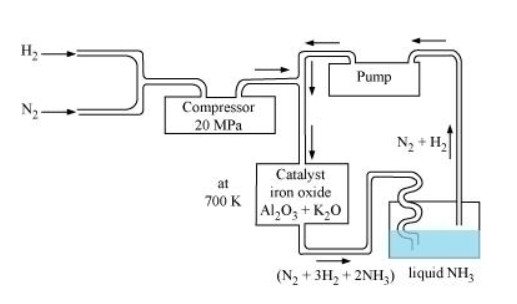Question 7: Illustrate how copper metal can give different products on reaction with $\mathrm{HNO}_{3}$.

Solution. Concentrated nitric acid is a strong oxidizing agent. It is used for oxidizing most metals. The products of oxidation depend on the concentration of the acid, temperature, and also on the material undergoing oxidation.

$3 \mathrm{Cu}+8 \mathrm{HNO}_{3(\text { dute })} \longrightarrow 3 \mathrm{Cu}\left(\mathrm{NO}_{3}\right)_{2}+2 \mathrm{NO}+4 \mathrm{H}_{2} \mathrm{O}$

$\mathrm{Cu}+4 \mathrm{HNO}_{3(\text { conc })} \longrightarrow \mathrm{Cu}\left(\mathrm{NO}_{3}\right)_{2}+2 \mathrm{NO}_{2}+2 \mathrm{H}_{2} \mathrm{O}$

Question 8: Give the resonating structures of $\mathrm{NO}_{2}$ and $\mathrm{N}_{2} \mathrm{O}_{5}$.

Solution. (1)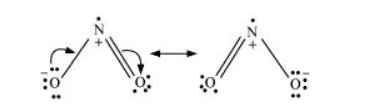(2)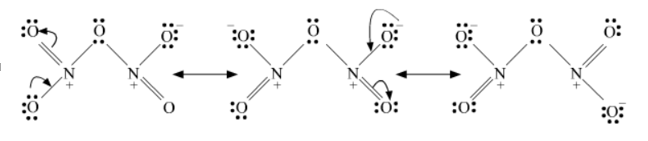Question 9: The HNH angle value is higher than HPH, HAsH and HSbH angles. Why? [Hint: Can be explained on the basis of sp $^{3}$ hybridisation in $\mathrm{NH}_{3}$ and only $s-p$ bonding between hydrogen and other elements of the group].

Solution. Hydride $\mathrm{NH}_{3} \mathrm{PH}_{3} \mathrm{AsH}_{3} \mathrm{SbH}_{3}$

$\mathrm{H}-\mathrm{M}-\mathrm{H}$ angle $107^{\circ} 92^{\circ} 91^{\circ} \mathrm{90}^{\circ}$

The above trend in the H-M-H bond angle can be explained on the basis of the electronegativity of the central atom. Since nitrogen is highly electronegative, there is high electron density around nitrogen. This causes greater repulsion between the electron pairs around nitrogen, resulting in maximum bond angle. We know that electronegativity decreases on moving down a group. Consequently, the repulsive interactions between the electron pairs decrease, thereby decreasing the $\mathrm{H}-\mathrm{M}-\mathrm{H}$ bond angle.

Question 10: Why does $\mathrm{R}_{3} \mathrm{P}=\mathrm{O}$ exist but $\mathrm{R}_{3} \mathrm{~N}=\mathrm{O}$ does not $(\mathrm{R}=$ alkyl group $)$ ?

Solution. $\mathrm{N}$ (unlike P) lacks the d-orbital. This restricts nitrogen to expand its coordination number beyond four. Hence, $\mathrm{R}_{3} \mathrm{~N}=\mathrm{O}$ does not exist.
Question 11: Explain why $\mathrm{NH}_{3}$ is basic while $\mathrm{BiH}_{3}$ is only feebly basic.

Solution. $\mathrm{NH}_{3}$ is distinctly basic while $\mathrm{BiH}_{3}$ is feebly basic.

Nitrogen has a small size due to which the lone pair of electrons is concentrated in a small region. This means that the charge density per unit volume is high. On moving down a group, the size of the central atom increases and the charge gets distributed over a large area decreasing the electron density. Hence, the electron donating capacity of group 15 element hydrides decreases on moving down the group

Question $12$ : Nitrogen exists as diatomic molecule and phosphorus as $P_{4}$. Why?

Solution. Nitrogen owing to its small size has a tendency to form p\pi-pm multiple bonds with itself. Nitrogen thus forms a very stable diatomic molecule, $\mathrm{N}_{2}$. On moving down a group, the tendency to form p\pi-pm bonds decreases (because of the large size of heavier elements). Therefore, phosphorus (like other heavier metals) exists in the $\mathrm{P}_{4}$ state.

Question 13: Write main differences between the properties of white phosphorus and red phosphorus.

Solution.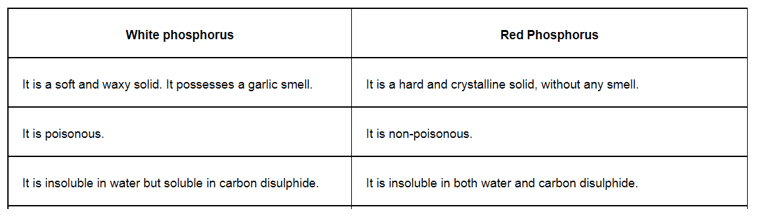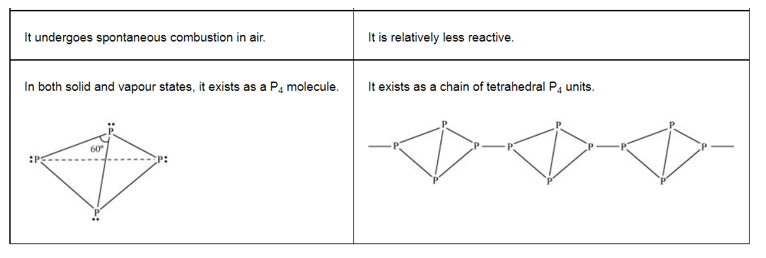Question 14: Why does nitrogen show catenation properties less than phosphorus?
Solution. Catenation is much more common in phosphorous compounds than in nitrogen compounds. This is because of the relative weakness of the N-N single bond as compared to the P-P single bond. Since nitrogen atom is smaller, there is greater repulsion of electron density of two nitrogen atoms, thereby weakening the $\mathrm{N}-\mathrm{N}$ single bond.

Question 15: Give the disproportionation reaction of $\mathrm{H}_{3} \mathrm{PO}_{3}$.

Solution. On heating, orthophosphorus acid $\left(\mathrm{H}_{3} \mathrm{PO}_{3}\right)$ disproportionates to give orthophosphoric acid $\left(\mathrm{H}_{3} \mathrm{PO}_{4}\right)$ and phosphine $\left(\mathrm{PH}_{3}\right)$. The oxidation states of $P$ in various species involved in the reaction are mentioned below.

$4 \mathrm{H}_{3} \mathrm{P} \mathrm{O}_{3} \longrightarrow 3 \mathrm{H}_{3} \stackrel{+3}{\mathrm{P}} \mathrm{O}_{4}+\mathrm{P}^{-3} \mathrm{H}_{3}$

Question $16$ : Can $\mathrm{PCl}_{5}$ act as an oxidising as well as a reducing agent? Justify.

Solution. $\mathrm{PCl}_{5}$ can only act as an oxidizing agent. The highest oxidation state that $\mathrm{P}$ can show is $+5 .$ In $\mathrm{PCl}_{5}$, phosphorus is in its highest oxidation state (+5). However, it can decrease its oxidation state and act as an oxidizing agent.

Question 17: Justify the placement of $O, S$, Se, Te and Po in the same group of the periodic table in terms of electronic configuration, oxidation state and hydride formation.

Solution. The elements of group 16 are collectively called chalcogens.

(i) Elements of group 16 have six valence electrons each. The general electronic configuration of these elements is $n s^{2} n p^{4}$, where $n$ varies from 2 to 6 .

(ii) Oxidation state:

As these elements have six valence electrons $\left(n s^{2} n p^{4}\right)$, they should display an oxidation state of $-2 .$ However, only oxygen predominantly shows the oxidation state of $-2$ owing to its high electronegativity. It also exhibits the oxidation state of $-1\left(\mathrm{H}_{2} \mathrm{O}_{2}\right)$, zero $\left(\mathrm{O}_{2}\right)$, and $+2\left(\mathrm{OF}_{2}\right)$. However, the stability of the $-2$ oxidation state decreases on moving down a group due to a decrease in the electronegativity of the elements. The heavier elements of the group show an oxidation state of $+2,+4$, and $+6$ due to the availability of $d$-orbitals.

(iii) Formation of hydrides:
These elements form hydrides of formula $\mathrm{H}_{2} \mathrm{E}$, where $\mathrm{E}=\mathrm{O}, \mathrm{S}$, Se, Te, PO. Oxygen and sulphur also form hydrides of type $\mathrm{H}_{2} \mathrm{E}_{2}$. These hydrides are quite volatile in nature.

Question 18: Why is dioxygen a gas but sulphur a solid?

Solution. Oxygen is smaller in size as compared to sulphur. Due to its smaller size, it can effectively form $p \pi-p \pi$ bonds and form $\mathrm{O}_{2}(\mathrm{O}==0)$ molecule. Also, the intermolecular forces in oxygen are weak van der Wall's, which cause it to exist as gas. On the other hand, sulphur does not form $\mathrm{M}_{2}$ molecule but exists as a puckered structure held together by strong covalent bonds. Hence, it is a solid.

Question 19: Knowing the electron gain enthalpy values for $\mathrm{O} \rightarrow \mathrm{O}^{-}$and $\mathrm{O} \rightarrow \mathrm{O}^{2-}$ as $-141$

and $702 \mathrm{~kJ} \mathrm{~mol}^{-1}$ respectively, how can you account for the formation of a

large number of oxides having $\mathrm{O}^{2-}$ species and $\mathrm{not} \mathrm{O}^{-}$?

(Hint: Consider lattice energy factor in the formation of compounds).

Solution. Stability of an ionic compound depends on its lattice energy. More the lattice energy of a compound, more stable it will be

Lattice energy is directly proportional to the charge carried by an ion. When a metal combines with oxygen, the lattice energy of the oxide involving $\mathrm{O}^{2-}$ ion is much more than the oxide involving $\mathrm{O}^{-}$ion. Hence, the oxide having $\mathrm{O}^{2-}$ ions are more stable than oxides having $\mathrm{O}^{-}$. Hence, we can say that formation of $\mathrm{O}^{2-}$ is energetically more favourable than formation of $\mathrm{O}^{-}$.

Question 20: Which aerosols deplete ozone?

Solution. Freons or chlorofluorocarbons (CFCs) are aerosols that accelerate the depletion of ozone. In the presence of ultraviolet radiations, molecules of CFCs break down to form chlorine-free radicals that combine with ozone to form oxygen.

Question 21: Describe the manufacture of $\mathrm{H}_{2} \mathrm{SO}_{4}$ by contact process?

Solution. Sulphuric acid is manufactured by the contact process. It involves the following steps:

Step (i):

Sulphur or sulphide ores are burnt in air to form $\mathrm{SO}_{2}$.

Step (ii):

By a reaction with oxygen, $\mathrm{SO}_{2}$ is converted into $\mathrm{SO}_{3}$ in the presence of $\mathrm{V}_{2} \mathrm{O}_{5}$ as a catalyst.

$2 \mathrm{SO}_{2(g)}+\mathrm{O}_{2(g)} \stackrel{\mathrm{v}_{2} \mathrm{O}_{3}}{\longrightarrow} 2 \mathrm{SO}_{3(\mathrm{~g})}$

Step (iii):

$\mathrm{SO}_{3}$ produced is absorbed on $\mathrm{H}_{2} \mathrm{SO}_{4}$ to give $\mathrm{H}_{2} \mathrm{~S}_{2} \mathrm{O}_{7}$ (oleum).

$\mathrm{SO}_{3}+\mathrm{H}_{2} \mathrm{SO}_{4} \longrightarrow \mathrm{H}_{2} \mathrm{~S}_{2} \mathrm{O}_{7}$

This oleum is then diluted to obtain $\mathrm{H}_{2} \mathrm{SO}_{4}$ of the desired concentration.

In practice, the plant is operated at 2 bar (pressure) and $720 \mathrm{~K}$ (temperature). The sulphuric acid thus obtained is $96-98 \%$ pure.

Question 22: How is $\mathrm{SO}_{2}$ an air pollutant?

Solution. Sulphur dioxide causes harm to the environment in many ways:

1. It combines with water vapour present in the atmosphere to form sulphuric acid. This causes acid rain. Acid rain damages soil, plants, and buildings, especially those made of marble.

2. Even in very low concentrations, $\mathrm{SO}_{2}$ causes irritation in the respiratory tract. It causes throat and eye irritation and can also affect the larynx to cause breathlessness.

3. It is extremely harmful to plants. Plants exposed to sulphur dioxide for a long time lose colour from their leaves. This condition is known as chlorosis. This happens because the formation of chlorophyll is affected by the presence of sulphur dioxide

Question $23:$ Why are halogens strong oxidising agents?

Solution. The general electronic configuration of halogens is $n p^{5}$, where $n=2-6 .$ Thus, halogens need only one more electron to complete their octet and to attain the stable noble gas configuration. Also, halogens are highly electronegative with low dissociation energies and high negative electron gain enthalpies. Therefore, they have a high tendency to gain an electron. Hence, they act as strong oxidizing agents.

Question 24: Explain why fluorine forms only one oxoacid, HOF.

Solution. Fluorine forms only one oxoacid i.e., HOF because of its high electronegativity and small size.
Question 25: Explain why inspite of nearly the same electronegativity, oxygen forms hydrogen bonding while chlorine does not.

Solution. Both chlorine and oxygen have almost the same electronegativity values, but chlorine rarely forms hydrogen bonding. This is because in comparison to chlorine, oxygen has a smaller size and as a result, a higher electron density per unit volume.

Question $26:$ Write two uses of $\mathrm{ClO}_{2}$.

Solution. Uses of $\mathrm{ClO}_{2}$ :

(i) It is used for purifying water.

(ii) It is used as a bleaching agent.

Question 27: Why are halogens coloured? Solution. Almost all halogens are coloured. This is because halogens absorb radiations in the visible region. This results in the excitation of valence electrons to a higher energy region. Since the amount of energy required for excitation differs for each halogen, each halogen displays a different colour.

Question 28: Write the reactions of $\mathrm{F}_{2}$ and $\mathrm{Cl}_{2}$ with water.

Solution.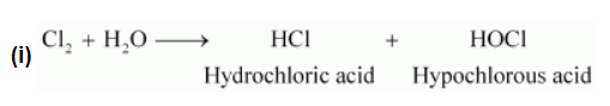(ii) $2 \mathrm{~F}_{2(g)}+2 \mathrm{H}_{2} \mathrm{O}_{(i)} \longrightarrow 4 \mathrm{H}^{+}_{(a q)}+4 \mathrm{~F}_{(a q)}^{-}+\mathrm{O}_{2(g)}+4 \mathrm{HF}_{(\alpha q)}$

Question $29$ : How can you prepare $\mathrm{Cl}_{2}$ from $\mathrm{HCl}$ and $\mathrm{HCl}$ from $\mathrm{Cl}_{2} ?$ Write reactions only.

Solution. (i) $\mathrm{Cl}_{2}$ can be prepared from $\mathrm{HCl}$ by Deacon's process.

$4 \mathrm{HCl}+\mathrm{O}_{2} \stackrel{\mathrm{CuCl}_{3}}{\longrightarrow} 2 \mathrm{Cl}_{2}+2 \mathrm{H}_{2} \mathrm{O}$

(ii) $\mathrm{HCl}$ can be prepared from $\mathrm{Cl}_{2}$ on treating it with water.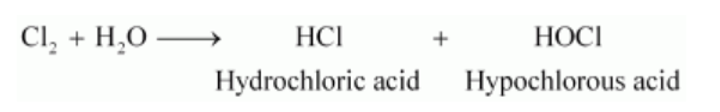Question 30: What inspired $\mathrm{N}$. Bartlett for carrying out reaction between $\mathrm{Xe}$ and $\mathrm{PtF}_{6}$ ?

Solution. Neil Bartlett initially carried out a reaction between oxygen and $\mathrm{PtF}_{6}$. This resulted in the formation of a red compound, $\mathrm{O}_{2}^{+}\left[\mathrm{PtF}_{6}\right]^{-}$

Later, he realized that the first ionization energy of oxygen $(1175 \mathrm{~kJ} / \mathrm{mol})$ and $\mathrm{Xe}(1170 \mathrm{~kJ} / \mathrm{mol})$ is almost the same. Thus, he tried to prepare a compound with $\mathrm{Xe}$ and $\mathrm{PtF}_{6}$. He was successful and a red-coloured compound, Xe $\left[\mathrm{PtF}_{6}\right]^{-}$was formed.

Question 31: What are the oxidation states of phosphorus in the following:

(ii) $\mathrm{PCl}_{3}$

(i) $\mathrm{H}_{3} \mathrm{PO}_{3}$

(iii) $\mathrm{Ca}_{3} \mathrm{P}_{2}$

(iv) $\mathrm{Na}_{3} \mathrm{PO}_{4}$

(v) $\mathrm{POF}_{3} ?$
Solution. Let the oxidation state of $p$ be $x$.

(i) $\mathrm{H}_{3} \mathrm{PO}_{3}$

$3+x+3(-2)=0$

$3+x-6=0$

$x-3=0$

$x=+3$

(ii) $\mathrm{PCl}_{3}$

$x+3(-1)=0$

$x-3=0$

$x=+3$

(iii) $\mathrm{Ca}_{3} \mathrm{P}_{2}$

$3(+2)+2(x)=0$

$6+2 x=0$

$2 x=-6$

$x=-3$

(iv) $\mathrm{Na}_{3} \mathrm{PO}_{4}$

$3(+1)+x+4(-2)=0$

$3+x-8=0$

$x-5=0$

$x=+5$

(v) $\mathrm{POF}_{3}$

$x+(-2)+3(-1)=0$

$x-5=0$

$x=+5$

Question 32: Write balanced equations for the following:

(i) $\mathrm{NaCl}$ is heated with sulphuric acid in the presence of $\mathrm{MnO}_{2}$.

(ii) Chlorine gas is passed into a solution of $\mathrm{Nal}$ in water.

Solution. (i) $4 \mathrm{NaCl}+\mathrm{MnO}_{2}+4 \mathrm{H}_{2} \mathrm{SO}_{4}$

$\longrightarrow \mathrm{MnCl}_{2}+4 \mathrm{NaHSO}_{4}+2 \mathrm{H}_{2} \mathrm{O}+\mathrm{Cl}_{2}$

(ii) $\mathrm{Cl}_{2}+\mathrm{NaI} \longrightarrow 2 \mathrm{NaCl}+\mathrm{I}_{2}$

Question 33: How are xenon fluorides $\mathrm{XeF}_{2}, \mathrm{XeF}_{4}$ and $\mathrm{XeF}_{6}$ obtained?

Solution. $\mathrm{XeF}_{2}, \mathrm{XeF}_{4,}$ and $\mathrm{XeF}_{6}$ are obtained by a direct reaction between $\mathrm{Xe}$ and $\mathrm{F} 2$. The condition under which the reaction is carried out determines the product.

$\mathrm{Xe}_{(g)}+\mathrm{F}_{2(g)} \quad \stackrel{673 \mathrm{~K}, \mathrm{lbar}}{\longrightarrow} \mathrm{XeF}_{2(s)}$

(Excess)

$\mathrm{Xe}_{(g)}+2 \mathrm{~F}_{2(g)} \stackrel{873 \mathrm{~K}, 7 \mathrm{bar}}{\longrightarrow} \mathrm{XeF}_{4(s)}$

$(1: 5$ ratio $)$

$\mathrm{Xe}_{(g)}+3 \mathrm{~F}_{2(g)} \quad \stackrel{573 \mathrm{~K}, 60-70 \mathrm{bar}}{\longrightarrow} \quad \mathrm{XeF}_{6(s)}$

$(1: 20$ ratio $)$

Question 34: With what neutral molecule is $\mathrm{ClO}^{-}$isoelectronic? Is that molecule a Lewis base?

Solution. $\mathrm{ClO}^{-}$is isoelectronic to CIF. Also, both species contain 26 electrons in all as shown.

Total electrons $\mathrm{ClO}^{-}=17+8+1=26$

In CIF $=17+9=26$

CIF acts like a Lewis base as it accepts electrons from $\mathrm{F}$ to form $\mathrm{CIF}_{3}$

Question 35: How are $\mathrm{XeO}_{3}$ and $\mathrm{XeOF}_{4}$ prepared?

Solution. (i) $\mathrm{XeO}_{3}$ can be prepared in two ways as shown.

$6 \mathrm{XeF}_{4}+12 \mathrm{H}_{2} \mathrm{O} \longrightarrow 4 \mathrm{Xe}+2 \mathrm{XeO}_{3}+24 \mathrm{HF}+3 \mathrm{O}_{2}$

$\mathrm{XeF}_{6}+3 \mathrm{H}_{2} \mathrm{O} \longrightarrow \mathrm{XeO}_{3}+6 \mathrm{HF}$

(ii) $\mathrm{XeOF}_{4}$ can be prepared using $\mathrm{XeF}_{6}$.

$\mathrm{XeF}_{6}+\mathrm{H}_{2} \mathrm{O} \longrightarrow \mathrm{XeOF}_{4}+2 \mathrm{HF}$

Question $36$ : Arrange the following in the order of property indicated for each set:

(i) $\mathrm{F}_{2}, \mathrm{Cl}_{2}, \mathrm{Br}_{2}, \mathrm{I}_{2}$ - increasing bond dissociation enthalpy.

(ii) $\mathrm{HF}, \mathrm{HCl}, \mathrm{HBr}, \mathrm{HI}$ - increasing acid strength.

(iii) $\mathrm{NH}_{3}, \mathrm{PH}_{3}, \mathrm{AsH}_{3}, \mathrm{SbH}_{3}, \mathrm{BiH}_{3}-$ increasing base strength.

Solution. (i) Bond dissociation energy usually decreases on moving down a group as the atomic size increases. However, the bond dissociation energy of $\mathrm{F} 2$ is lower than that of $\mathrm{Cl} 2$ and Br2. This is due to the small atomic size of fluorine. Thus, the increasing order for bond dissociation energy among halogens is as follows:

$\mathrm{I}_{2}<\mathrm{F}_{2}<\mathrm{Br}_{2}<\mathrm{Cl}_{2}$

(ii) $\mathrm{HF}<\mathrm{HCl}<\mathrm{HBr}<\mathrm{HI}$

The bond dissociation energy of $\mathrm{H}-\mathrm{X}$ molecules where $\mathrm{X}=\mathrm{F}, \mathrm{Cl}, \mathrm{Br}, \mathrm{l}$, decreases with an increase in the atomic size. Since $\mathrm{H}$-I bond is the weakest, $\mathrm{Hl}$ is the strongest acid.

(iii) $\mathrm{BiH}_{3} \leq \mathrm{SbH}_{3}<\mathrm{AsH}_{3}<\mathrm{PH}_{3}<\mathrm{NH}_{3}$

On moving from nitrogen to bismuth, the size of the atom increases while the electron density on the atom decreases. Thus, the basic strength decreases.

Question $37:$ Which one of the following does not exist?

Solution. (i) $\mathrm{XeOF}_{4}$

(ii) $\mathrm{NeF}_{2}$

(iii) $\mathrm{XeF}_{2}(\mathrm {iv}) \mathrm{XeF}_{6}$

Solution. $\mathrm{Ne} \mathrm{F}_{2}$ does not exist.

Question 38: Give the formula and describe the structure of a noble gas species which is isostructural with:

(i) $\mathrm{ICl}_{4}^{-}$

(ii) $\mathrm{IBr}_{2}^{-}$

(iii) $\mathrm{BrO}_{3}^{-}$

Solution. (i)

$\mathrm{XeF}_{4}$ is isoelectronic with $\mathrm{ICl}_{4}^{-}$and has square planar geometry.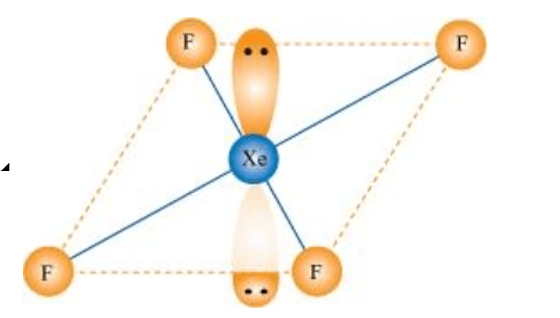(ii) $\mathrm{XeF}_{2}$ is isoelectronic to $\mathrm{IBr}_{2}^{-}$and has a linear structure.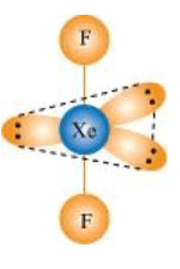(iii) $\mathrm{XeO}_{3}$ is isostructural to $\mathrm{Br} \mathrm{O}_{3}^{-}$and has a pyramidal molecular structure.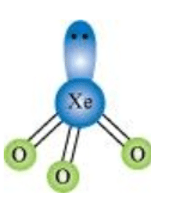Question 39: Why do noble gases have comparatively large atomic sizes?

Solution. Noble gases do not form molecules. In case of noble gases, the atomic radii corresponds to van der Waal's radii. On the other hand, the atomic radii of other elements correspond to their covalent radii. By definition, van der Waal's radii are larger than covalent radii. It is for this reason that noble gases are very large in size as compared to other atoms belonging to the same period.

Question 40: List the uses of Neon and argon gases

Solution. Uses of neon gas:

(i) It is mixed with helium to protect electrical equipments from high voltage.

(ii) It is filled in discharge tubes with characteristic colours.

(iii) It is used in beacon lights.

Uses of Argon gas:

(i) Argon along with nitrogen is used in gas-filled electric lamps. This is because Ar is more inert than N.

(ii) It is usually used to provide an inert temperature in a high metallurgical process.

(iii) It is also used in laboratories to handle air-sensitive substances.